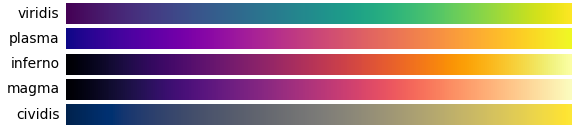## Matplotlib configuration

Images in BornAgain examples are generated using the Python library Matplotlib.

Default settings can be overridden by by function arguments, Matplotlib ressources, or environment variables.

### Plot invocation

When running BornAgain through Python sripts, Matplotlib is invoked either directly, or indirectly through BornAgain functions like run_and_plot. For direct invocation, see the Matplotlib documentation. Here we are concerned with indirect invocation through standard BornAgain plot functions.

The functions run_and_plot, plot_simulation_result, and a number of lower-level functions, are all implemented in the Python module plot_utils that is part of the bornagain module. These functions support the keyword arguments

• intensity_min,
• intensity_max,
• xlabel,
• ylabel,
• zlabel,
• cmap: heat map, see below,
• aspect: aspect ratio, default is ‘auto’, other possible values are ’equal’, or a number,
• noshow: if True, run Matplotlib in batch mode, without displaying a plot.

So if an example script contains the code line

    ba.run_and_plot()


then the plot can be modified through keyword arguments like

    ba.run_and_plot(cmap='jet', intensity_min=2e-8, intensity_max=2)


### Matplotlib resources

Matplotlib default settings can be changed through resource arguments or resource files. See the Matplotlib customizing tutorial for an introduction to resources; see in particular the section on matplotlibrc files. Note, however, that the heat map (cmap) resource setting is ignored when Matplotlib is used via BornAgain plot functions.

### Environment variables

The following environment variables can be used to control BornAgain’s plot behavior:

• NOSHOW, to prevent plot routines from opening a plot window; for use in tests and other batch processing;
• CMAP, the default heat map, see below;
• USETEX, to use the original LaTeX text processor (USETEX=ON), see below.

## Heat map

Whenever plotting is done through plot_simulation_result, or through one of the other functions from plot_utils.py, BornAgain imposes its default color scheme “inferno”. Inferno is one of the five “perceptually uniform sequential” color schemes recommended in the Matplotlib color schemes tutorial.Note that our choice of “inferno” is hard-coded, and overrides the CMAP environment variable. It can however be overridden by a keyword argument, like cmap='jet'.

## LaTeX markup

Text enclosed between dollar signs $...$ is interpreted as LaTeX markup. It allows us to plot greek letters ($\alpha$), superscripts ($F^2$), subscripts ($\phi_{\rm i}$), and special symbols ($90^\circ$).

By default, it is processed by Matplotlib’s own LaTeX processor. By activating Matplotlib’s rc variable usetex, this can be overridden so that the original LaTeX text processor is used, and labels are typeset in LaTeX’s computer modern fonts.

For usetex mode to work, it may be necessary to install extra software. Under Debian, the required packages are texlive-latex-extra (and dependencies), dvipng and cm-super-minimal.

Original LaTeX fonts differ in many respects from Matplotlib’s default Helvetica fonts. Fonts from these different families should not be mixed. Therefore, in usetex mode it is important that all plot labels and titles be enclosed in $...$, or for upright text ${\rm ...}$.